Integral of a Derivative

INTRODUCTION

In calculus, integrals and derivatives are operators which means they are shorthand for more complicated expressions involving limits.  We define the integral operator on the function f(x) as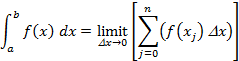where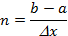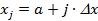And for the derivative operator on the function f(x) is defined as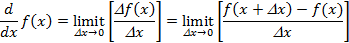COMBINATION OF OPERATORS

Unfortunately in the case of a derivative, the notation is often misinterpreted as a real fraction.  And even worse, sometimes the misinterpretation results in a simplification that gives the correct answer.    But since this serendipitous happenstance is not always correct, it behooves us to look at the expressions in greater detail.   The correct interpretation of the integral of a function Z(x) multiplied by its derivative is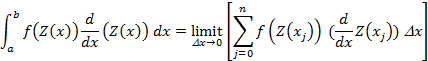Please note that the term “dx” does not simply “cancel out”.  Rather we write the derivative in terms as its definition as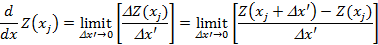Combining expressions note that the two limits contain the terms Δx and Δx’ which both approach zero in the limit.   Since we can approach zero in both terms by any route including the convenient one of setting them equal to each other, we do so and can write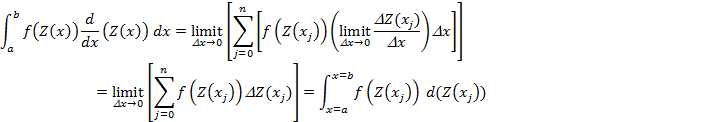EXAMPLE

By analogy we can define an inverse function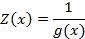So thatwhere ln(z) = loge(z).